# RS Aggarwal Solutions for Class 6 Maths Chapter 20 Two-Dimensional Reflection Symmetry (Linear Symmetry)

RS Aggarwal Solutions for Class 6 Maths Chapter 20 Two-Dimensional Reflection Symmetry (Linear Symmetry) are given here in PDF format. RS Aggarwal Solutions help the students to understand the chapter thoroughly clearing all their doubts which they come across while solving problems. By meticulously practicing RS Aggarwal textbook solutions, students ace the examinations.

Mathematics experts at BYJU’S have solved RS Aggarwal textbook questions with thorough solutions which are easy to understand by students. Download PDF of Class 6 Chapter 20 in their respective links.

## Download PDF of RS Aggarwal Solutions for Class 6 Chapter 20 Two-Dimensional Reflection Symmetry (Linear Symmetry)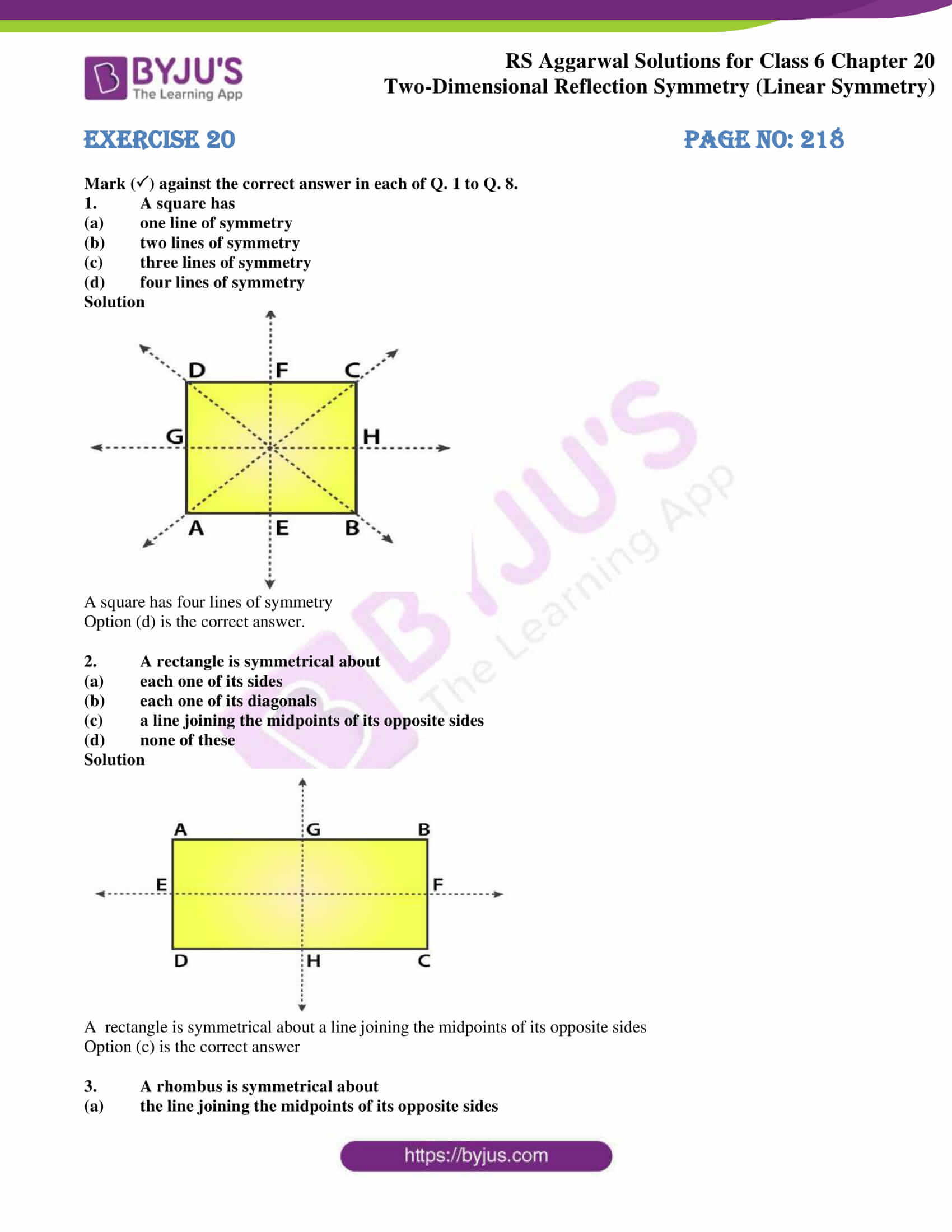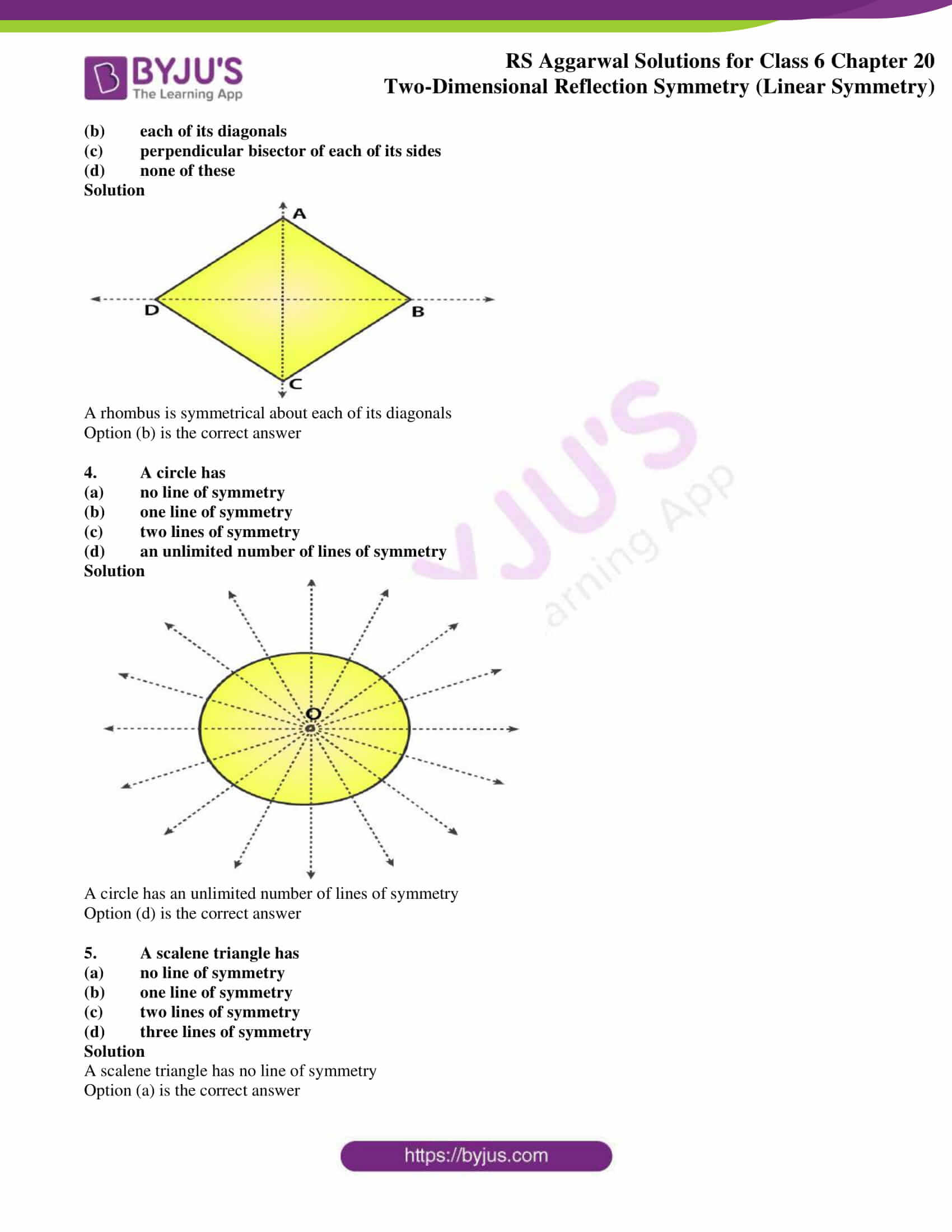### Access answers of RS Aggarwal Solutions for Class 6 Maths Chapter 20 Two-Dimensional Reflection Symmetry (Linear Symmetry)

Exercise 20 PAGE NO: 218

Mark () against the correct answer in each of Q. 1 to Q. 8.

1. A square has

(a) one line of symmetry

(b) two lines of symmetry

(c) three lines of symmetry

(d) four lines of symmetry

Solution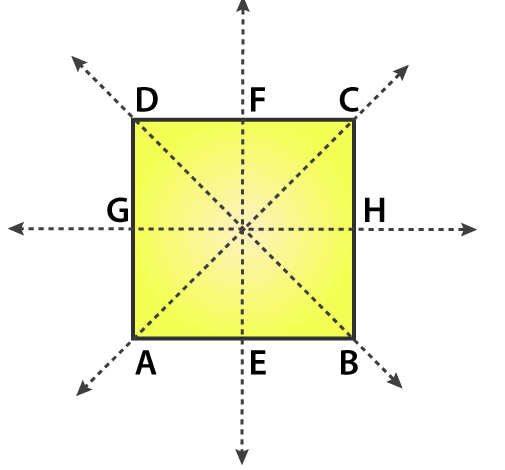A square has four lines of symmetry

Option (d) is the correct answer.

2. A rectangle is symmetrical about

(a) each one of its sides

(b) each one of its diagonals

(c) a line joining the midpoints of its opposite sides

(d) none of these

Solution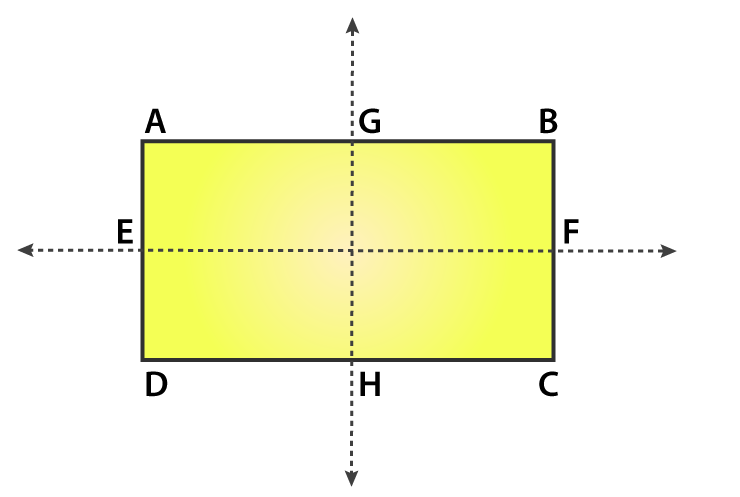A rectangle is symmetrical about a line joining the midpoints of its opposite sides

Option (c) is the correct answer

3. A rhombus is symmetrical about

(a) the line joining the midpoints of its opposite sides

(b) each of its diagonals

(c) perpendicular bisector of each of its sides

(d) none of these

Solution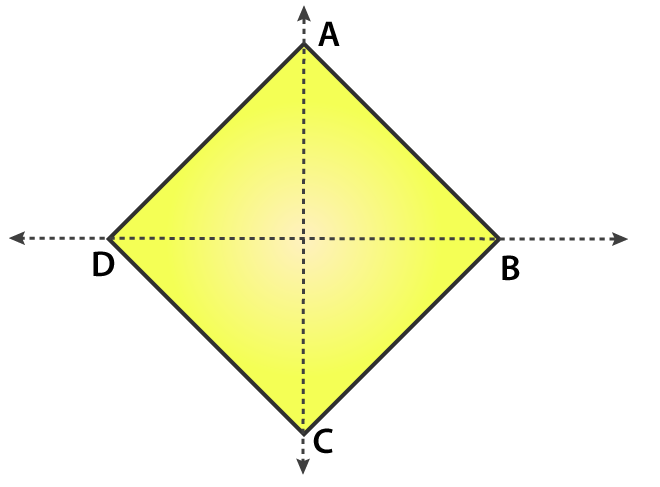A rhombus is symmetrical about each of its diagonals

Option (b) is the correct answer

4. A circle has

(a) no line of symmetry

(b) one line of symmetry

(c) two lines of symmetry

(d) an unlimited number of lines of symmetry

Solution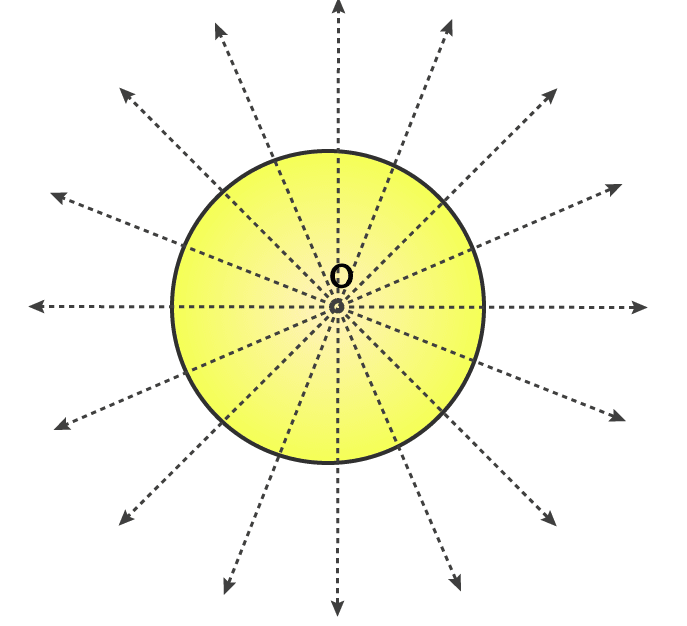A circle has an unlimited number of lines of symmetry

Option (d) is the correct answer

5. A scalene triangle has

(a) no line of symmetry

(b) one line of symmetry

(c) two lines of symmetry

(d) three lines of symmetry

Solution

A scalene triangle has no line of symmetry

Option (a) is the correct answer

### RS Aggarwal Solutions for Class 6 Maths Chapter 20 Two-Dimensional Reflection Symmetry (Linear Symmetry)

Chapter 20 has 1 exercise. All questions from this chapter of RS Aggarwal textbook have been solved in detail. Let’s have a look at the topic which is mentioned here

• Linear Symmetry

A figure is said to be symmetrical about a line l, if it is identical on either side of l, it is called Linear Symmetry.